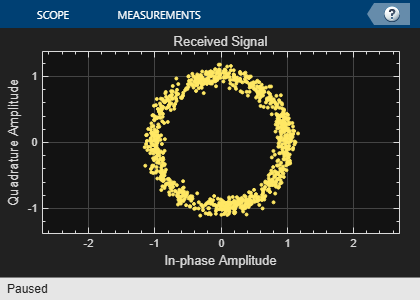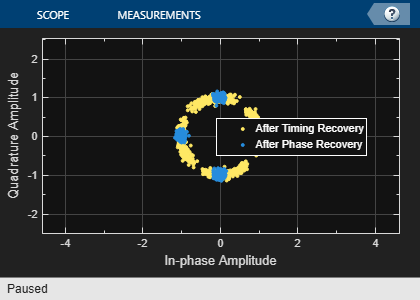Main Content

# MSK Signal Recovery

Model channel impairments such as timing phase offset, carrier frequency offset, and carrier phase offset for a minimum shift keying (MSK) signal. Use `comm.MSKTimingSynchronizer` and `comm.CarrierSynchronizer` System objects to synchronize such signals at the receiver. The MSK timing synchronizer recovers the timing offset, while a carrier synchronizer recovers the carrier frequency and phase offsets.

Initialize system variables by running the MATLAB script `configureMSKSignalRecoveryEx`. Define the logical control variable `recoverTimingPhase` to enable timing phase recovery, and `recoverCarrier` to enable carrier frequency and phase recovery.

```configureMSKSignalRecoveryEx; recoverTimingPhase = true; recoverCarrier = true; ```

### Modeling Channel Impairments

Specify the sample delay, `timingOffset`, that the channel model applies. Create a variable fractional delay object to introduce the timing delay to the transmitted signal.

```timingOffset = 0.2; varDelay = dsp.VariableFractionalDelay; ```

Create a `comm.PhaseFrequencyOffset` System object to introduce carrier phase and frequency offsets to a modulated signal. Because the MSK modulator upsamples the transmitted symbols, set the `SampleRate` property to the ratio of the `samplesPerSymbol` and the sample time, `Ts`.

```freqOffset = 50; phaseOffset = 30; pfo = comm.PhaseFrequencyOffset(... 'FrequencyOffset',freqOffset, ... 'PhaseOffset',phaseOffset, ... 'SampleRate',samplesPerSymbol/Ts); ```

Create a `comm.AWGNChannel` System object to add white Gaussian noise to the modulated signal. The noise power is determined by the `EbNo` property, that is the bit energy to noise power spectral density ratio. Because the MSK modulator generates symbols with 1 Watt of power, set the signal power property of the AWGN channel System object to 1.

```EbNo = 20 + 10*log10(samplesPerSymbol); chAWGN = comm.AWGNChannel(... 'NoiseMethod','Signal to noise ratio (Eb/No)', ... 'EbNo',EbNo,... 'SignalPower',1, ... 'SamplesPerSymbol',samplesPerSymbol); ```

### Timing Phase, Carrier Frequency, and Carrier Phase Synchronization

Create an MSK timing synchronizer to recover symbol timing phase using a fourth-order nonlinearity method.

```timeSync = comm.MSKTimingSynchronizer(... 'SamplesPerSymbol',samplesPerSymbol, ... 'ErrorUpdateGain',0.02); ```

Create a carrier synchronizer to recover both carrier frequency and phase. Because the MSK constellation is QPSK with a 0-degree phase offset, set the `comm.CarrierSynchronizer` accordingly.

```phaseSync = comm.CarrierSynchronizer(... 'Modulation','QPSK', ... 'ModulationPhaseOffset','Custom', ... 'CustomPhaseOffset',0, ... 'SamplesPerSymbol',1); ```

### Stream Processing Loop

The simulation modulates data using MSK modulation. The modulated symbols pass through the channel model, which applies timing delay, carrier frequency and phase shift, and additive white Gaussian noise. The receiver performs timing phase and carrier frequency and phase recovery. Finally, the signal symbols are demodulated and the bit error rate is calculated. The `plotResultsMSKSignalRecoveryEx` script generates scatter plots in this order to show these effects:

1. Channel impairments

2. Timing synchronization

3. Carrier synchronization

At the end of the simulation, the example displays the timing phase, frequency, and phase estimates as a function of simulation time.

```for p = 1:numFrames %------------------------------------------------------------------------ % Generate and modulate data %------------------------------------------------------------------------ txBits = randi([0 1],samplesPerFrame,1); txSym = modem(txBits); %------------------------------------------------------------------------ % Transmit through channel %------------------------------------------------------------------------ % % Add timing offset rxSigTimingOff = varDelay(txSym,timingOffset*samplesPerSymbol); % % Add carrier frequency and phase offset rxSigCFO = pfo(rxSigTimingOff); % % Pass the signal through an AWGN channel rxSig = chAWGN(rxSigCFO); % % Save the transmitted signal for plotting plot_rx = rxSig; % %------------------------------------------------------------------------ % Timing recovery %------------------------------------------------------------------------ if recoverTimingPhase % Recover symbol timing phase using fourth-order nonlinearity % method [rxSym,timEst] = timeSync(rxSig); % Calculate the timing delay estimate for each sample timEst = timEst(1)/samplesPerSymbol; else % Do not apply timing recovery and simply downsample the received % signal rxSym = downsample(rxSig,samplesPerSymbol); timEst = 0; end % Save the timing synchronized received signal for plotting plot_rxTimeSync = rxSym; %------------------------------------------------------------------------ % Carrier frequency and phase recovery %------------------------------------------------------------------------ if recoverCarrier % The following script applies carrier frequency and phase recovery % using a second order phase-locked loop (PLL), and removes phase ambiguity [rxSym,phEst] = phaseSync(rxSym); removePhaseAmbiguityMSKSignalRecoveryEx; freqShiftEst = mean(diff(phEst)/(Ts*2*pi)); phEst = mod(mean(phEst),360); % in degrees else freqShiftEst = 0; phEst = 0; end % Save the phase synchronized received signal for plotting plot_rxPhSync = rxSym; %------------------------------------------------------------------------ % Demodulate the received symbols %------------------------------------------------------------------------ rxBits = demod(rxSym); %------------------------------------------------------------------------ % Calculate the bit error rate %------------------------------------------------------------------------ errorStats = BERCalc(txBits,rxBits); %------------------------------------------------------------------------ % Plot results %------------------------------------------------------------------------ plotResultsMSKSignalRecoveryEx; end ```Display the bit error rate and the total number of symbols processed by the error rate calculator.

```BitErrorRate = errorStats(1) TotalNumberOfSymbols = errorStats(3) ```
```BitErrorRate = 4.0001e-06 TotalNumberOfSymbols = 499982 ```

### Conclusion and Further Experimentation

The recovery algorithms are demonstrated by using constellation plots taken after timing, carrier frequency, and carrier phase synchronization.

Open the script to create a writable copy of this example and its supporting files. Then, to show the effects of the recovery algorithms, you can enable and disable the logical control variables `recoverTimingPhase` and `recoverCarrier` and rerun the simulation.

### Appendix

This example uses these scripts:

• `configureMSKSignalRecoveryEx`

• `plotResultsMSKSignalRecoveryEx`

• `removePhaseAmbiguityMSKSignalRecoveryEx`

## Support

#### Bridging Wireless Communications Design and Testing with MATLAB

Download white paper